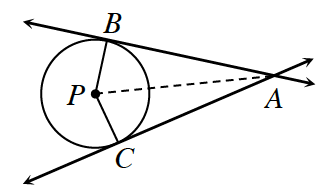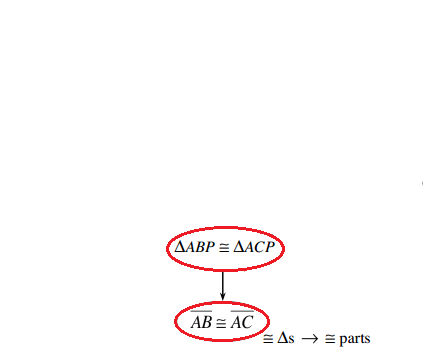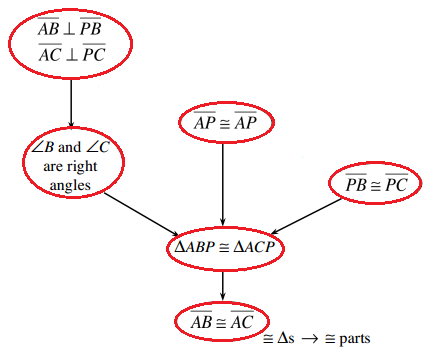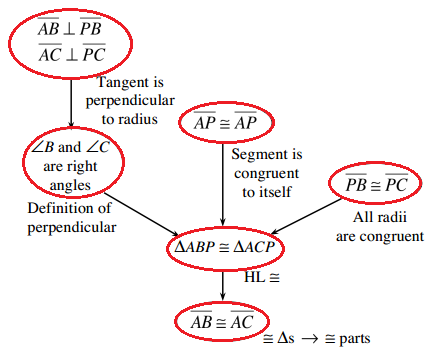### Home > GC > Chapter 11 > Lesson 11.1.2 > Problem11-25

11-25.Prove that when two lines that are tangent to the same circle intersect, the lengths between the point of intersection and the points of tangency are equal. That is, in the diagram below, if $\overleftrightarrow{ A B }$ is tangent to $⊙P$ at $B$, and $\overleftrightarrow{ A C }$ is tangent to $⊙P$ at $C$, prove that ${AB} = {AC}$. Use either a flowchart or a two-column proof.

Prove that $ΔABP≅ΔACP$.

Recall the triangle congruence shortcuts you know.

First, decide if the triangles are congruent.
Which congruency shortcut did you use?

Write your conclusion in an oval near the bottom.
Below right, write the shortcut used. 2 bubbles, with arrow from top to bottom bubble, labeled as follows: top, triangle, A, b, p, congruent to triangle, a, c, p, bottom, segment, a, b, congruent to segment, a, c, and congruent triangles give congruent parts.

If the triangles are congruent, then the lengths are the same. 4 bubbles added above 2 previous bubbles, #1 with arrow to #2, with arrow to top previous bubble, & bubbles #3 & #4 each with arrow to top previous bubble. Labels as follows: #1: Segment, a, b, perpendicular, to segment, p, b, segment, a, c, perpendicular to segment, p, c. #2: angle, b, and angle, c, are right angles. #3: segment, a, p, congruent to segment, a, p. #4: segment, p, b, congruent to segment, p, c.

Write the reasons you knew the triangle to be congruent. Additional labels added outside the following bubbles: #1: tangent is perpendicular to radius. #2, definition of perpendicular. #3, segment is congruent to itself. #4, All radii are congruent. Original top bubble: h, l, congruency.